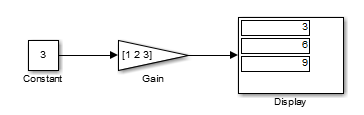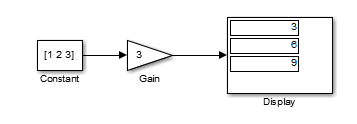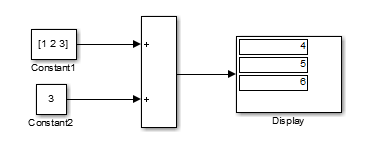## Determine Signal Dimensions

Simulink® blocks can output one-dimensional, two-dimensional, or multidimensional signals. The Simulink user interface and documentation generally refer to 1-D signals as vectors and 2-D or multidimensional signals as matrices. A one-element array is frequently referred to as a scalar. A row vector is a 2-D array that has one row. A column vector is a 2-D array that has one column.

• A one-dimensional (1-D) signal consists of a series of one-dimensional arrays output at a frequency of one array (vector) per simulation time step.

• A two-dimensional (2-D) signal consists of a series of two-dimensional arrays output at a frequency of one 2-D array (matrix) per block sample time.

• A multidimensional signal consists of a series of multidimensional (two or more dimensions) arrays output at a frequency of one array per block sample time. You can specify multidimensional arrays with any valid MATLAB® multidimensional expression, such as [4 3]. See Multidimensional Arrays for information on multidimensional arrays.

Simulink blocks vary in the dimensionality of the signals they can accept or output. Some blocks can accept or output signals of any dimension. Some can accept or output only scalar or vector signals.

Note

Simulink does not support dynamic signal dimensions during a simulation. That is, the dimension of a signal must remain constant while a simulation is executing. However, you can change the size of a signal during a simulation. See Variable-Size Signal Basics.

If a block can emit nonscalar signals, the dimensions of the signals that the block outputs depend on the block parameters, if the block is a source block; otherwise, the output dimensions depend on the dimensions of the block input and parameters.

To determine the dimensions that a signal ultimately uses for simulation, first update the block diagram (for example, by pressing Ctrl+D). Then, choose one of these techniques:

• Display the dimensions directly on the block diagram. Use this technique to trace signal dimensions along a path of blocks. In the model, on the Debug tab, select Information Overlays > Signal Dimensions).

• Inspect the dimensions in the Model Data Editor, which shows you information in a searchable, sortable table. In the table, the right side of each cell in the Dimensions column shows the true dimensions of the corresponding signal line in the model.

### Simulink Blocks that Support Multidimensional Signals

The Simulink Block Data Type Support table includes a column identifying the blocks with multi-dimension signal support.

1. In the MATLAB command line, enter `showblockdatatypetable`.

A separate window with the Simulink Block Data Type Support table opens.

2. In the Block column, locate the name of a Simulink block. Columns to the right are data types or features. An a X in a column indicates support for that feature.

Simulink supports signals with up to 32 dimensions. Do not use signals with more than 32 dimensions.

### Determine the Output Dimensions of Source Blocks

A source block is a block that has no inputs. Examples of source blocks include the Constant block and the Sine Wave block. See Sources for a complete listing of Simulink source blocks. The output dimensions of a source block are the same as those of its output value parameters if the block Interpret vector parameters as 1-D parameter is off (that is, not selected in the block parameter dialog box). If the Interpret vector parameters as 1-D parameter is on, the output dimensions equal the output value parameter dimensions unless the parameter dimensions are N-by-1 or 1-by-N. In the latter case, the block outputs a vector signal of width N.

As an example of how the output value parameters and Interpret vector parameters as 1-D parameter of a source block determine the dimensionality of its output, consider the Constant block. This block outputs a constant signal equal to its Constant value parameter. The following table illustrates how the dimensionality of the Constant value parameter and the setting of the Interpret vector parameters as 1-D parameter determine the dimensionality of the block output.

Constant ValueInterpret vector parameters as 1-DOutput

scalar

off

one-element array

scalar

on

one-element array

1-by-N matrix

off

1-by-N matrix

1-by-N matrix

on

N-element vector

N-by-1 matrix

off

N-by-1 matrix

N-by-1 matrix

on

N-element vector

M-by-N matrix

off

M-by-N matrix

M-by-N matrix

on

M-by-N matrix

Simulink source blocks allow you either to specify the dimensions of the signals that they output or specify values from which Simulink infers the dimensions. You can therefore use the source blocks to introduce signals of various dimensions into your model.

### Determine the Output Dimensions of Nonsource Blocks

If a block has inputs, the dimensions of its outputs are, after scalar expansion, the same as those of its inputs. (All inputs must have the same dimensions, as discussed in Signal and Parameter Dimension Rules).

### Signal and Parameter Dimension Rules

When creating a Simulink model, you must observe the following rules regarding signal and parameter dimensions.

#### Input Signal Dimension Rule

All nonscalar inputs to a block must have the same dimensions.

A block can have a mix of scalar and nonscalar inputs as long as all the nonscalar inputs have the same dimensions. Simulink expands the scalar inputs to have the same dimensions as the nonscalar inputs (see Scalar Expansion of Inputs and Parameters).

#### Block Parameter Dimension Rule

In general, block parameters must have the same dimensions as the dimensions of the inputs to the block. Simulink performs some processing that provides flexibility relating to that general rule.

• A block can have scalar parameters corresponding to nonscalar inputs. In this case, Simulink expands a scalar parameter to have the same dimensions as the corresponding input (see Scalar Expansion of Inputs and Parameters).

• If an input is a vector, the corresponding parameter can be either an N-by-1 or a 1-by-N matrix. In this case, Simulink applies the N matrix elements to the corresponding elements of the input vector. This exception allows use of MATLAB row or column vectors, which are actually 1-by-N or N-by-1 matrices, respectively, to specify parameters that apply to vector inputs.

#### Vector or Matrix Input Conversion Rules

Simulink converts vectors to row or column matrices and row or column matrices to vectors under the following circumstances:

• If a vector signal is connected to an input that requires a matrix, Simulink converts the vector to a one-row or one-column matrix.

• If a one-column or one-row matrix is connected to an input that requires a vector, Simulink converts the matrix to a vector.

• If the inputs to a block consist of a mixture of vectors and matrices and the matrix inputs all have one column or one row, Simulink converts the vectors to matrices having one column or one row, respectively.

Note

You can configure Simulink to display a warning or error message if a vector or matrix conversion occurs during a simulation. See Vector/matrix block input conversion for more information.

### Scalar Expansion of Inputs and Parameters

Scalar expansion is the conversion of a scalar value into a nonscalar array. Many Simulink blocks support scalar expansion of inputs and parameters. Block-specific descriptions indicate whether Simulink applies scalar expansion to block inputs and parameters.

Scalar expansion of inputs refers to the expansion of scalar inputs to match the dimensions of other nonscalar inputs or nonscalar parameters. When the input to a block is a mix of scalar and nonscalar signals, Simulink expands the scalar inputs into nonscalar signals having the same dimensions as the other nonscalar inputs. For example, a scalar of 4 is expanded to the vector [4 4 4] if the associated nonscalar has a dimension of 3.

Scalar expansion of parameters refers to the expansion of scalar block parameters to match the dimensions of nonscalar inputs.

Input(s)Associated Block ParameterScalar Expansion

Scalar

Nonscalar

Input expanded to match parameter dimensions.

Nonscalar

Scalar

Scalar parameter expanded to match number of elements of input.

Combination of scalar and nonscalar

No corresponding parameter

Scalar inputs expanded to match dimensions of largest nonscalar input.

#### Scalar Input and Nonscalar Parameter

In this example, the Constant block input to the Gain block is scalar. The Gain block Gain parameter is a nonscalar. Simulink expands the scalar input to match the dimensions of a nonscalar Gain parameter, as reflected in the simulation results in the Display block.#### Nonscalar Input and Scalar Parameter

In this example, the Constant block input to the Gain block is nonscalar. The Gain block Gain parameter is a scalar. Simulink expands the scalar parameter to match the dimensions of a nonscalar input from the Constant block, as reflected in the simulation results in the Display block.#### Scalar and Nonscalar Inputs and No Associated Parameter

In this example, the Constant1 block input to the Sum block is nonscalar, and the Constant2 block input is scalar. The Sum block has no associated parameter. Simulink expands the scalar input from Constant2 to match to the dimensions of the nonscalar Constant1 block input. The input is expanded to the vector `[3 3 3]`.#### Get Compiled Port Dimensions

To get the dimensions of port signals, pause the simulation by using the button. Select a block and use the `PortHandles` parameter. Then, use the `GetCompiledPortDimensions` parameter. For example, if you step forward in this model and select the Constant block:```ph = get_param(gcb,'PortHandles'); dim = get_param(ph.Outport,'CompiledPortDimensions')```
```dim = 2 2 3```

For nonbus ports, the result is an array in which the first element is the number of dimensions (in this case, `2`), and the next two elements (corresponding to the number of dimensions) are the values of the dimensions.

For buses, the results include some extra elements. Suppose that you step forward in a model with buses, and you select the Bus Creator block that contains a nested bus:```ph = get_param(gcb,'PortHandles'); dim = get_param(ph.Outport,'CompiledPortDimensions')```
`dim = -2 4 1 2 1 1 3 4 5 6 5 2 3 4 5 6 `

The first element is `-2`, which indicates that the port corresponds to a virtual bus. The second element is the number of leaf nodes (`4`). The subsequent elements follow the same pattern as for nonbus signals.

• The output port of the first leaf node has one dimension (`1`), and the value in that dimension is `2`.

• The output port of the second leaf node has one dimension (`1`), and the value in that dimension is `1`.

• The output port of the third leaf node has three dimensions (`3`), and the values in those dimensions are `4 5 6`.

• The output port of the fourth leaf node has five dimensions (`5`) and the values in those dimensions are `2 3 4 5 6`.

When querying dimensions, nonvirtual buses are treated as scalars. Suppose the nested bus in the previous example were a nonvirtual bus instead of a virtual bus.```ph = get_param(gcb,'PortHandles'); dim = get_param(ph.Outport,'CompiledPortDimensions')```
`dim = -2 3 1 2 1 1 1 1`

The second element indicates three leaf nodes instead of four leaf nodes because the nonvirtual bus is treated as a scalar. The third leaf node corresponds to the Bus Creator block that outputs the nonvirtual bus. The output port of the third leaf node has one dimension (`1`), and the value in that dimension is `1`.